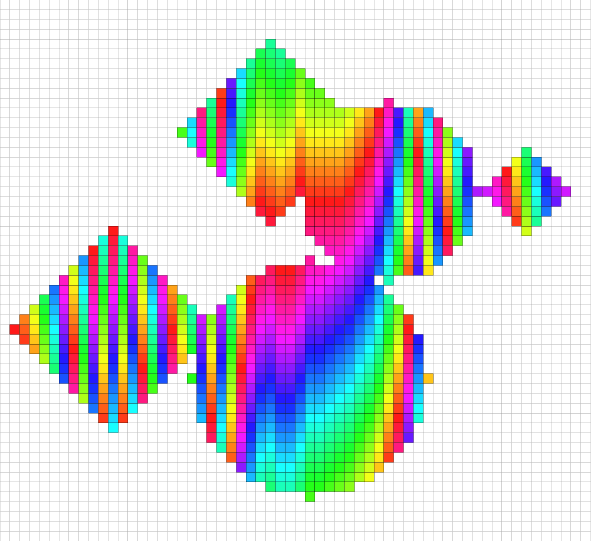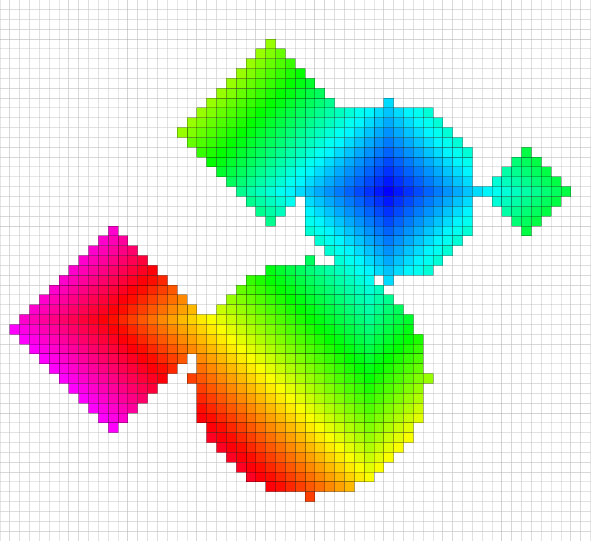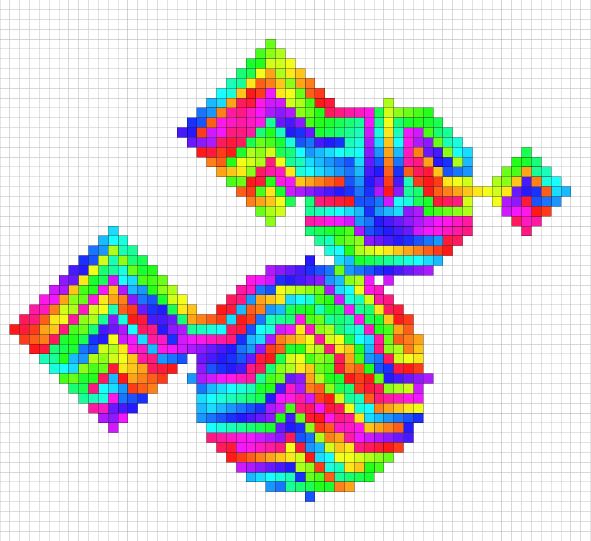DGtal  1.3.beta
Basic graph definitions and concepts
Author(s) of this documentation:
by Jacques-Olivier Lachaud

Part of the Graph package.

This module defines what are graphs in DGtal, as well as basic associated concepts. Relevant digital models of graph are notably found in Topology package.

The following programs are related to this documentation: graph/graphTraversal.cpp, graph/volDistanceTraversal.cpp

# Graph concepts in DGtal

There are many different ways for representing a given graph, even without considering types that attached data to vertices and edges, the most notable ones are adjacency lists per vertex, incidence matrix, outgoing edges per vertex. A rather comprehensive set of finite graph concepts may for instance be found in the boost::graph module.

Graphs in DGtal may be finite (like Object) or only locally finite (like MetricAdjacency). Graphs in DGtal are undirected. Therefore, they are two different concepts for graphs:

All graphs define a Vertex (which must be instance-able, copy-able, assignable) and a Size type (type for counting vertices). Furthermore, they must provide a default type VertexSet for representing a set of Vertex, and a defaut type rebinder VertexMap for representing a map with key Vertex.

Note
DGtal graph concepts are very light compared to boost::graph for instance. The advantage is that it is easy to create new models of graph in DGtal. The disadvantage is that you may not be able to design the best optimized graph algorithms in some case.
Interfacing with boost::graph library
Note
For now, DGtal does not provide a generic graph model.

# Using graphs

This section gathers the basic ways of using graphs.

## Instanciating graphs

The instanciation of graphs (like any object) is dependent on the chosen graph model. For instance, to instantiate a MetricAdjacency or an Object, you can consult Digital topology and digital objects. To instantiate a DigitalSurface, you may consult High-level classes for digital surfaces.

Here is a simple example for creating a 2D digital object seen with the (4,8) adjacency (in example graph/graphTraversal.cpp)

using namespace Z2i;
Point p1( -41, -36 ), p2( 18, 18 );
Domain domain( p1, p2 );
DigitalSet shape_set( domain );
Shapes<Domain>::addNorm2Ball( shape_set, Point( -2, -1 ), 9 );
Shapes<Domain>::addNorm1Ball( shape_set, Point( -14, 5 ), 9 );
Shapes<Domain>::addNorm1Ball( shape_set, Point( -30, -15 ), 10 );
Shapes<Domain>::addNorm2Ball( shape_set, Point( -10, -20 ), 12 );
Shapes<Domain>::addNorm1Ball( shape_set, Point( 12, -1 ), 4 );
Object4_8 g( dt4_8, shape_set ); // 4-adjacency graph.
typedef Object4_8 Graph;
typedef Point Vertex;
BOOST_CONCEPT_ASSERT(( concepts::CUndirectedSimpleGraph< Graph > ));

The last line above checks that Object are finite graphs.

## Enumerating vertices of a graph

We simply use the range [begin(),end()) offered by graphs. This is done as follows.

int n = 0;
for ( Graph::ConstIterator it = g.begin(), itEnd = g.end();
it != itEnd; ++it, ++n )
{ // Vertex are colored in order.
Vertex vtx = *it;
board << CustomStyle( specificStyle,
new CustomColors( Color::Black,
cmap_hue( n ) ) )
<< vtx;
}
board.saveEPS("graphTraversal-enum.eps");Coloring vertices of an object graph according to the enumeration order.

## Enumerating edges of a graph

You may obtain edges of a graph by using method writeNeighbors as follows:

int nn = 0;
int mm = 0;
std::vector<Vertex> neighbors;
for ( Graph::ConstIterator it = g.begin(), itEnd = g.end();
it != itEnd; ++it, ++nn )
{
Vertex vtx = *it;
std::back_insert_iterator< std::vector<Vertex> > neighIt
= std::back_inserter( neighbors );
g.writeNeighbors( neighIt, vtx );
mm += neighbors.size();
neighbors.clear();
}
trace.info() << "Graph has " << nn << " vertices and "
<< (mm/2) << " edges." << std::endl;

## Graph traversal with visitors

Sometimes it is more convenient to traverse in a specific order, and not in the arbitrary order given by vertex enumeration. That is exactly the purpose of visitors, specified by concept concepts::CGraphVisitor. Visitors traverse the graph or only subparts of the graph by adjacencies. In some sense, they trace a tree from the initial seed. A vertex is visited at most once. Data may be associated to each visited vertex, like its distance to the initial seed. Three models of visitors are provided, two of them implement the classical breadth-first and depth-first traversal:

• model BreadthFirstVisitor performs the traversal of a connected graph by breadth-first search. If the graph is not connected, only the connected component(s) containing the initial seed(s) are visited. This visitor also computes the distance to the initial seed(s), which is also the topological distance to the seed(s) (see graph/graphTraversal.cpp).
• model DepthFirstVisitor performs the traversal of a connected graph by depth-first search. If the graph is not connected, only the connected component(s) containing the initial seed(s) are visited. This visitor also computes the distance to the initial seed(s) as the number of ancestors in the depth search (see graph/graphTraversal.cpp).
• model DistanceBreadthFirstVisitor performs the traversal of a connected graph by a modified breadth-first search: it is based on a priority queue, the priority of which is given by a distance object (and not by the topological distance as in classical breadth-first traversal). If the graph is not connected, only the connected component(s) containing the initial seed(s) are visited. This visitor also gives the distance given by the distance object (see graph/volDistanceTraversal.cpp).

The snippet below shows how to use a visitor to color vertices according to the topological distance to the initial seed (the point (-2,-1)). The node is a std::pair<Vertex,Data>, where Data is the distance.

typedef BFSVisitor::Node Node;
BFSVisitor bfv( g, Point( -2, -1 ) );
while( ! bfv.finished() )
{ // Vertex are colored according to the distance to initial seed.
Node node = bfv.current();
board << CustomStyle( specificStyle,
new CustomColors( Color::Black,
<< node.first; // the vertex
bfv.expand();
}
board.saveEPS("graphTraversal-bfs.eps");Coloring vertices of an object graph according to the topological distance to a seed (breadth-first traversal).
Note
The traversal of the graph can be changed dynamically in two ways:

## Transforming a visitor into a range

It is sometimes convenient to transform a visitor into the usual range / iterator pair. For instance, it is used by light containers for digital surfaces to iterate through all vertices on-the-fly (see LightImplicitDigitalSurface, LightExplicitDigitalSurface). This is done easily with class GraphVisitorRange. This class transforms an arbitary model of concepts::CGraphVisitor into a single pass input range (on Vertex or on Node).

The following snippet transforms a DepthFirstVisitor into a range.

typedef DepthFirstVisitor<Graph, std::set<Vertex> > DFSVisitor;
typedef GraphVisitorRange<DFSVisitor> VisitorRange;
VisitorRange range( new DFSVisitor( g, Point( -2, -1 ) ) );
n = 0;
for ( VisitorRange::ConstIterator it = range.begin(), itEnd = range.end();
it != itEnd; ++it, ++n )
{ // Vertex are colored according to their order (depth first order here).
Vertex vtx = *it;
board << CustomStyle( specificStyle,
new CustomColors( Color::Black,
cmap_hue( n ) ) )
<< vtx;
}
board.saveEPS("graphTraversal-dfs-range.eps");Coloring vertices of an object graph according to their order given by a depth-first traversal.
ConstIterator
MyDigitalSurface::ConstIterator ConstIterator
Definition: greedy-plane-segmentation-ex2.cpp:93
DGtal::HyperRectDomain< Space >
DGtal::Z2i::Point
Space::Point Point
Definition: StdDefs.h:95
DGtal::trace
Trace trace
Definition: Common.h:154
DGtal::Color::Black
static const Color Black
Definition: Color.h:413
Vertex
TriMesh::Vertex Vertex
Definition: testTriangulatedSurface.cpp:57
DGtal::Trace::info
std::ostream & info()
DGtal::Z2i::dt4_8
static const DT4_8 dt4_8
Definition: StdDefs.h:113
DGtal::Z2i::Object4_8
Object< DT4_8, DigitalSet > Object4_8
Definition: StdDefs.h:101